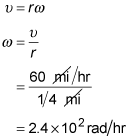##### Physics I: 501 Practice Problems For Dummies (+ Free Online Practice)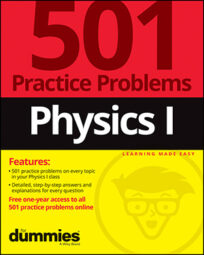In physics, you can combine angular velocity with radian measurement to calculate the distance and speed of a moving object. For example, you can calculate the angular speed of a car as it travels along a curve.

Here are some practice questions that you can try.

## Practice questions

1. The moon rotates once on its axis in about 27 days. If the moon's radius is 1,734 kilometers, how far in kilometers does an astronaut standing on the moon's surface move in 1 day?

2. You drive along the freeway at 60 miles per hour. You go through a curve with a radius of one quarter mile. What is your angular speed in radians per hour in this curve?

The following are the answers to the practice questions:

1.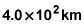The angular speed of the moon is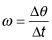For this problem,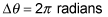(one complete revolution) and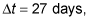so the angular speed is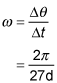To find the angle through which the astronaut rotates in one day, solve the equation above for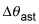(the subscript ast means that this is the angle through which the astronaut rotates in one day, not the angle through which the moon rotates in 27 days). The result is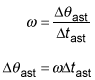where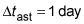is the time during which the astronaut rotates. Insert the known quantities to find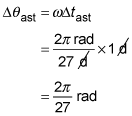To find the distance that the astronaut travels, use the equation relating arc length to arc radius and the angle subtended by the arc: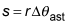Use r = 1,734 kilometers to find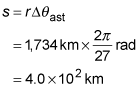2.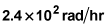The angular speed is related to the tangential velocity and the radius of curvature by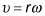Solve this for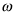and insert the known quantities of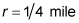and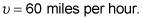The result is